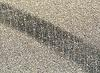# Determining Vehicle Speed from Yaw MarksWhen a vehicle is in yaw it is rotating around a vertical axis through it's center of mass. The long, curved Y shaped marks left by the tires on the pavement were characteristic of this motion. If they were striated and of constantly diminishing radius they were of great interest for collision reconstruction because the vehicle's speed could be determined from them.

A series of chord and middle ordinate measurements were made of the outside front tire mark at regular intervals starting where the outside rear tire started to track outside of the path of the front tire. These measurements were used to calculated the radius of the curved tire mark and the series was compared to insure that the radius was continually getting smaller. If so, this was a true yaw and combined with the co-efficient of friction for the road surface, the vehicle speed could be calculated.

For the simplest case, a flat road, the equation speed equals 11.27 times the square root of the front tire mark radius multiplied by the co-efficient of friction of the road surface would yield the speed of the vehicle at that point of the yaw in km/h.

This equation and the method of measuring the yaw was exhaustively tested when I was a collision analyst. We would gather for training sessions, usually on an airstrip somewhere, and one of the senior reconstructionists would induce a yaw in our test vehicle while we measured speed with radar. The outcome of our calculations agreed closely with the measured speed.